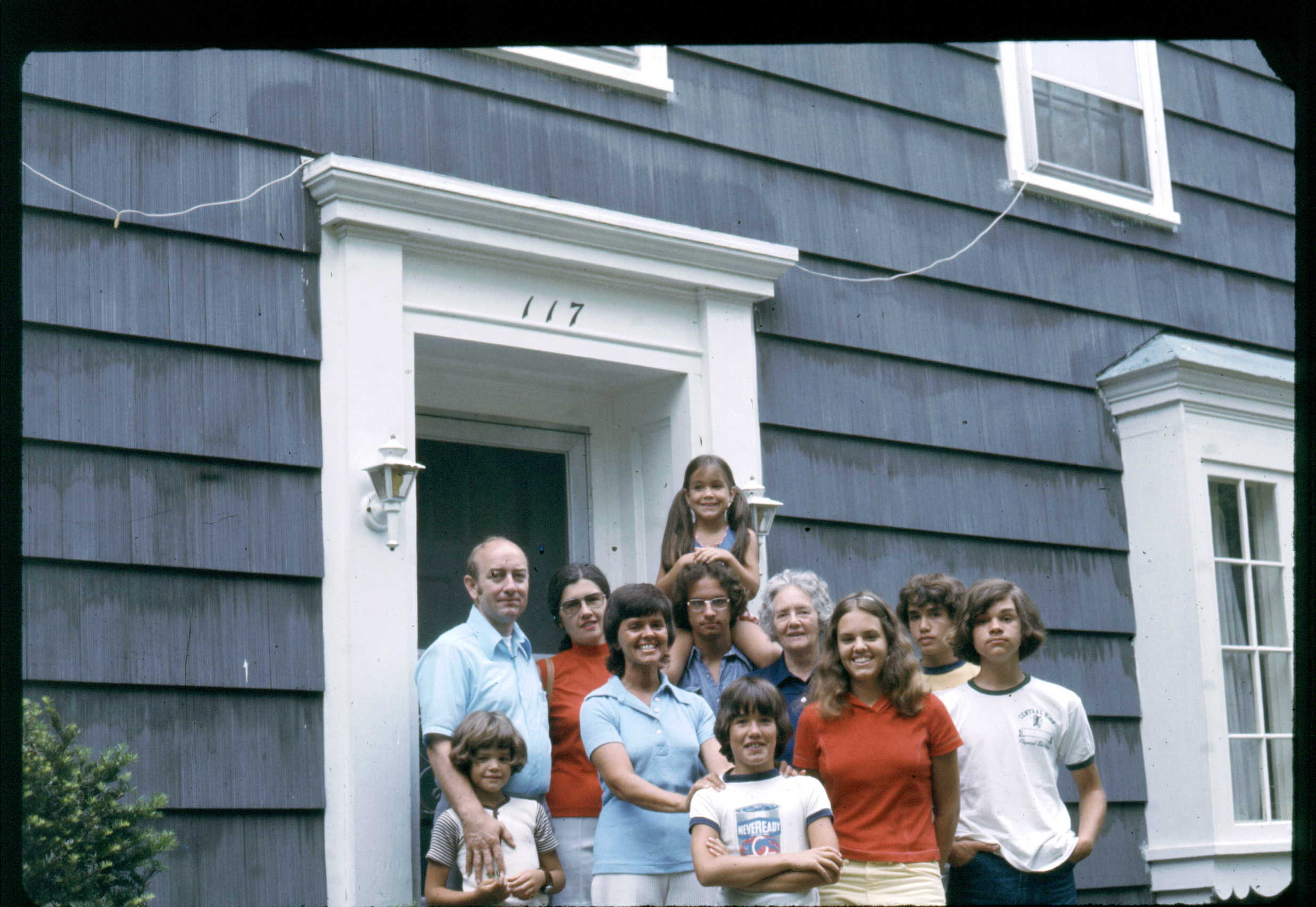## Goulet and Graff Families

Place mouse over face for identification of personDescription Goulet and Graff Families Date / Time 1975-08-14 Country / State / County / City / Placename USA, NH, xx, Manchester Persons (t-b,l-r) Elizabeth Anne Graff, Leo Christopher Graff, Nannette Marie Graff, Jerome Thomas Goulet, Donald Christopher Graff, James Augustine Goulet, Steven Noah Goulet, Winnifred Anne Goulet, Florence Agnes Goulet, René Therese Goulet, Michael Graff
```lib card:
dc:
title 		=	Goulet and Graff Families
creator 	=	Jerome Goulet
subject 	=
description 	=	Original (scanned analog or digital), names to faces, stored to LibCard "format", within image exif:
publisher 	=	Jerome Goulet
contributor 	=
date 		=	1975-08-14
resourcetype 	=
iformat 	=
if_mime 	=	image/jpeg
if_resolution 	=	1800x1800
if_dimensions 	=
if_dim_x 	=	2472
if_dim_y 	=	1704
resourceID 	=
source 		=	photograph (analog or digital)
language 	=	eng
rights 		=	Copyright 2020, Jerome Goulet<jtgoulet@yahoo.com>, GNU General Public License
lc:
when:
dateUTC		=	1975-08-14
timeUTC		=	0000
tz		=	+0000

dateLocal	=	1975-08-14
timeLocal	=	0000
where:
admin0		=	USA
admin1		=	NH
admin2		=	xx
admin3		=	Manchester
pName		=	x
lat		=	x
lon		=	x
who:
person:
name:
surName		=	Graff
givenName	=	Elizabeth Anne
aka		=	EAG
relation	=
pip:	(person in picture)
boxX		=	1281
boxY		=	874
boxWidth	=	101
boxHeight	=	101
label		=
uri		=
person:
name:
surName		=	Graff
givenName	=	Leo Christopher
aka		=	LCG
relation	=
pip:	(person in picture)
boxX		=	883
boxY		=	1027
boxWidth	=	136
boxHeight	=	136
label		=
uri		=
person:
name:
surName		=	Graff
givenName	=	Nannette Marie
aka		=	NMG1961
relation	=
pip:	(person in picture)
boxX		=	1041
boxY		=	1088
boxWidth	=	112
boxHeight	=	112
label		=
uri		=
person:
name:
surName		=	Goulet
givenName	=	Jerome Thomas
aka		=	JTG
relation	=
pip:	(person in picture)
boxX		=	1277
boxY		=	1091
boxWidth	=	108
boxHeight	=	108
label		=
uri		=
person:
name:
surName		=	Graff
givenName	=	Donald Christopher
aka		=	DCG
relation	=
pip:	(person in picture)
boxX		=	1690
boxY		=	1124
boxWidth	=	96
boxHeight	=	96
label		=
uri		=
person:
name:
surName		=	Goulet
givenName	=	James Augustine
aka		=	JAG
relation	=
pip:	(person in picture)
boxX		=	1811
boxY		=	1116
boxWidth	=	118
boxHeight	=	118
label		=
uri		=
person:
name:
surName		=	Goulet
givenName	=	Steven Noah
aka		=	SNG
relation	=
pip:	(person in picture)
boxX		=	879
boxY		=	1388
boxWidth	=	97
boxHeight	=	97
label		=
uri		=
person:
name:
surName		=	Goulet
givenName	=	Winnifred Anne
aka		=	WAG
relation	=
pip:	(person in picture)
boxX		=	1158
boxY		=	1131
boxWidth	=	112
boxHeight	=	112
label		=
uri		=
person:
name:
surName		=	Goulet
givenName	=	Florence Agnes
aka		=	FAG1954
relation	=
pip:	(person in picture)
boxX		=	1439
boxY		=	1111
boxWidth	=	104
boxHeight	=	104
label		=
uri		=
person:
name:
surName		=	Goulet
givenName	=	René Therese
aka		=	RTG
relation	=
pip:	(person in picture)
boxX		=	1557
boxY		=	1150
boxWidth	=	118
boxHeight	=	118
label		=
uri		=
person:
name:
surName		=	Graff
givenName	=	Michael
aka		=	MG
relation	=
pip:	(person in picture)
boxX		=	1357
boxY		=	1305
boxWidth	=	122
boxHeight	=	122
label		=
uri		=
what:
eventName	=	Goulet and Graff Families
```